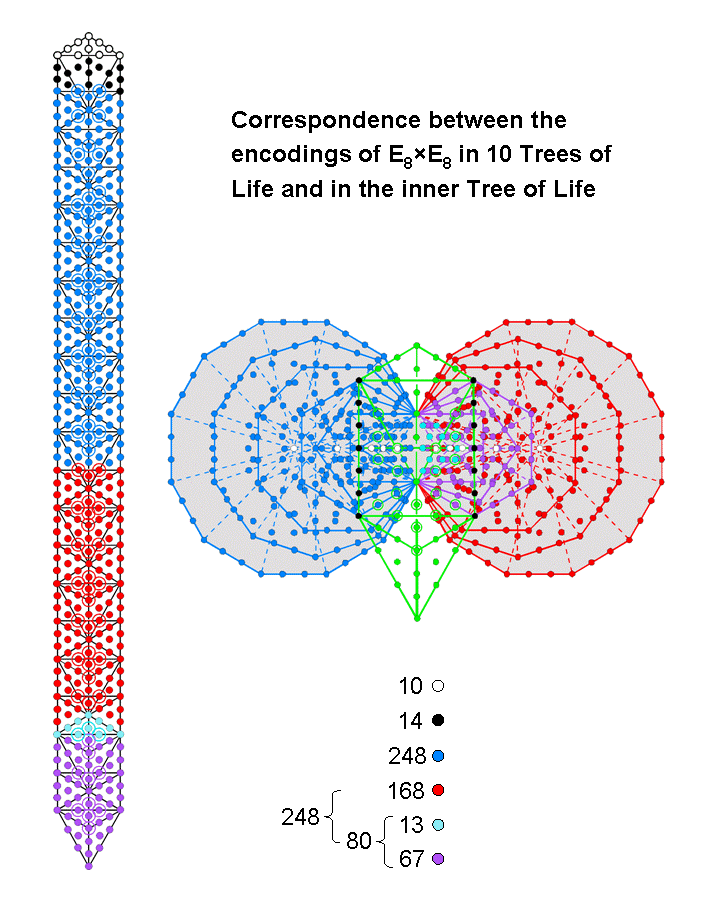<< Previous    1...   25  26    28  29  ...56    Next >>

#27 Correspondence between the encodings of E8×E8 in 10 Trees of Life & in the inner Tree of LifeWe saw in #24 that the 520 yods outside the root edge of the (7+7) enfolded polygons correspond to the 520 yods in 10 overlapping Trees of Life. Each Tree represents one of the 10 dimensions of the space-time of superstrings. The 496 spin-1 particles that transmit their forces are symbolized by the 496 yods up to (but not including) Chesed of the tenth Tree. There are 248 yods up to Chesed of the fifth Tree (the 31st SL). They symbolize the 248 spin-1 particles that transmit the forces between superstrings of ordinary matter governed by the first of the two E8 Lie groups in E8×E8. The remaining 248 blue yods above the 31st SL symbolize the 248 particles that transmit the forces between superstrings of shadow matter governed by the second E8 group. The lowest Tree has 80 yods, where 80 is the number of Yesod. They comprise 13 turquoise yods down to the Path between Chokmah and Binah and 67 violet yods below this Path, where 67 is the number value of Binah. Above the 1-tree are 168 red yods up to Chesed of the fifth Tree. This 80:168 division of the dimension 248 of E8 is a characteristic feature of holistic systems embodying this number (see The holistic pattern).

As one corner of each triangle coincides with the centre of a hexagon and as one corner of each pentagon coincides with the centre of a decagon, only five of each set of seven polygons have centres that are not also corners of polygons. The (7+7) enfolded polygons have 524 yods. (524−5−5=514) yods surround these ten white centres. The 14 enfolded polygons lie in the plane containing the side pillars of the outer Tree of Life. The latter has 70 yods, of which seven coloured black lie on each side pillar, leaving 60 green yods. The number of yods in the (7+7) enfolded polygons that surround the ten pure centres and which are unshared with the outer Tree = 514 − 7 − 7 = 500 = 50×10. ELOHIM, the Godname of Binah with number value 50, prescribes those yods intrinsic to the inner Tree of Life that surround centres of its polygons. Four yods lie in the root edge, so that (500−4=496) intrinsic yods outside the root edge surround the ten centres. Each set of seven enfolded polygons contains 248 such yods. This is how the inner Tree of Life encodes the root composition of E8×E8, one of the two symmetry groups governing the forces between heterotic superstrings. The factor group arises from the two mirror-image halves of the 14 enfolded polygons of the inner Tree of Life.

The following table shows the numbers of intrinsic yods outside the root edge that surround the centres of each enfolded polygon:

 Triangle Square Pentagon Hexagon Octagon Decagon Dodecagon Total Number of corners 0 2 3 2 6 8 10 31 Number of hexagonal yods 3+10=13 4+14=18 5+18=23 5+14=19 8+30=38 10+38=48 12+46=58 47+170=217 Number of yods 13 20 26 21 44 56 68 248 67 168 80

("m+n" means that the polygon has m hexagonal yods at the centres of its sectors and n hexagonal yods on their sides).

The first four enfolded polygons have 80 yods that correspond to the 80 yods in the 1-tree and the last three enfolded polygons have 168 yods that correspond to the 168 red yods above them up to the 31st SL. The 80:168 division appears naturally in the seven enfolded polygons. Moreover, the division of the 80 yods into the 13 turquoise yods and the 67 violet yods appears in the first four enfolded polygons, the triangle having 13 turquoise yods (all hexagonal yods) and the square, pentagon & hexagon having 67 violet yods. Notice also that the 248 intrinsic yods surrounding the five independent centres consist of 31 corners of polygons and 217 hexagonal yods. This compares with the 31 SLs (that is, 31 corners of tetractyses) and the 217 hexagonal yods up to Chesed of the fifth Tree. It is highly implausible that such a degree of correlation between two objects claimed to embody the group-theoretical number 248 could be due to chance. Instead, it is powerful evidence that they are isomorphic, sacred-geometrical embeddings of this archetypal number.

According to the table, the enfolded decagon and dodecagon have (38+46=84) hexagonal yods on the sides of their 22 tetractyses, leaving 84 yods in the octagon and in these two polygons. This 84:84 division is characteristic of holistic systems that embody the number 168 (see The holistic pattern). As explained in many other discussions, it physically manifests as the 840:840 division of circular turns in the outer and inner 2½ spirals made by each helical whorl of the UPA/subquark superstring as it winds around its axis of spin. Every half-revolution of a helical whorl contains 168 turns and every quarter-revolution contains 84 turns.

 << Previous    1...   25  26    28  29  ...56    Next >>

Home